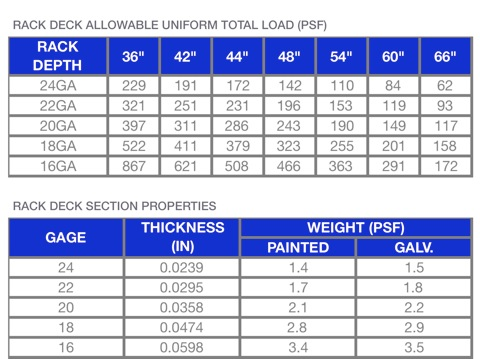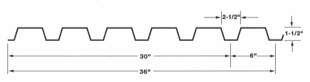Example Calculation:

A pallet rack level is 48” deep x 96” wide. What would be the lightest gage of rack deck allowable to achieve a uniform storage level capacity of 5,000 pounds (LBS)?

Step 1 Determine the area (SF) of the storage level
48” x 96” = 4,608 SQ IN = 32 SF

Step 2 Divide the required capacity (5,000 LBS) by the area of the storage level (32 SF) to obtain the total load
5,000 LBS/32 SF = 156.3 LBS/SF = 156.3 PSF

Step 3 Find the lowest number above 156.3 PSF in the 48” column and match the gage

Solution: 196 PSF = 22GA RACK DECK